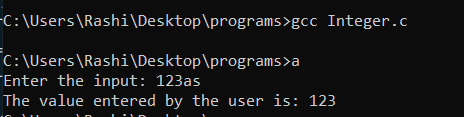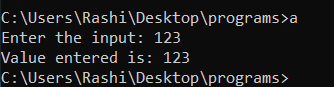Related Articles

# Program that allows integer input only

• Difficulty Level : Basic
• Last Updated : 21 Jul, 2021

Given an input value N, the task is to allow taking only integer input from the user.

Now, if the user enters any input other than an integer, that is, a character or symbol, it will not be accepted by the program. Below is the C program to implement this approach:

## C

 `// C program for the above approach``#include `` ` `// Driver Code``int` `main()``{``    ``int` `a;``    ``printf``(``"Enter the input: "``);`` ` `    ``// Take the input from console``    ``scanf``(``"%d"``, &a);`` ` `    ``// Display the output``    ``printf``(``"The value entered by the user is: "``);``    ``printf``(``"%d"``, a);`` ` `    ``return` `0;``}`

Output:Explanation: In the above program, if the input is a character value, then the scanf() function will take the input but will not print anything on the output screen or it will ignore the character and print the integers only. So, to avoid this problem, let’s discuss how to write a program that takes only integer input only. Below are the steps for the same:

Steps: The steps involved in the program below are:

1. Step 1: The user creates a function called getIntegeronly() and assigns it to int x.
2. Step 2: In this function, the input which is entered will go through a do-while loop in which the if statement checks the ASCII code of the integer. If the ASCII code matches the integer ASCII code, the input will be seen on the screen.
```do
{
If( ch>=48 && ch<=57)
}

Here ch is the input to be enter and 48 to 57 is the
ASCII Code of 0 to 9 respectively. ```
• Step 3: To make the single number a digit, multiply the number and add the integer that is entered.

num = num * 10 + (ch – 48)
Here num * 10 will change the place value of the integer to make
it digit and (ch – 48) will add the integer by subtracting its ASCII code.

• Step 4: To break the loop an if statement is added so that the loop does not go in an infinite loop.
```if(ch == 13)
break;

Here 13 is the ASCII code of carriage return which breaks and
return the input value.```
• Step 5: The return function returns the integer stored in num to x and the output gets printed on the screen.
`printf("you have entered %d", x)`

Below is the C program to implement the above approach:

## C

 `// C program for the above approach``#include `` ` `// Driver Code``int` `main()``{``    ``int` `x = 0;``    ``x = getIntegerOnly();``    ``printf``(``"\nvalue entered is: %d"``, x);``}`` ` `// Function to check if the user``// entered value is integer or not``int` `getIntegerOnly()``{``    ``int` `num = 0, ch;``    ``printf``(``"Enter the input: "``);``    ``do` `{``        ``ch = getch();`` ` `        ``// Checks the ASCII code of '``        ``// digits 0 to 9``        ``if` `(ch >= 48 && ch <= 57) {``            ``printf``(``"%c"``, ch);`` ` `            ``// To make a digit``            ``num = num * 10 + (ch - 48);``        ``}`` ` `        ``// 13 is carriage return it breaks``        ``// and return the input``        ``if` `(ch == 13)``            ``break``;``    ``} ``while` `(1);`` ` `    ``return` `(num);``}`

Output:Explanation: It can be observed while executing the program, that the program is only accepting the integer input and printing the input on the screen.

Want to learn from the best curated videos and practice problems, check out the C Foundation Course for Basic to Advanced C.

My Personal Notes arrow_drop_up